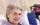# Aunt

Annie aunt is 35 years old, Annie is 14 years. For how many years will aunt have twice as many years as Annie?

Result

t =  7

#### Solution:

35+t = 2(14+t)

t = 7

t = 7

Calculated by our simple equation calculator.

Leave us a comment of example and its solution (i.e. if it is still somewhat unclear...):Be the first to comment!#### To solve this verbal math problem are needed these knowledge from mathematics:

Do you have a linear equation or system of equations and looking for its solution? Or do you have quadratic equation?

## Next similar examples:

1. How oldThe student who asked how many years he answered: "After 10 years I will be twice as old than as I was four years ago. How old is student?
2. FamilyMom is 39 years old. Karl is eight years and Paul 4 years. For many years mother would old just like two children together?
3. Six yearsIn six years Jan will be twice as old as he was six years ago. How old is he?
4. CakesGrandmother baked cakes. Half of its was poppy, quarter with plum jam and 16 cheesecakes. How many cakes she baked in total?
5. Forest nurseryIn the forest nursery after winter, they found that 1/10 stems died out of them. For them, they land 193 new spruces. How many spruces are in the forest nursery?
6. NormThree workers planted 3555 seedlings of tomatoes in one dey. First worked at the standard norm, the second planted 120 seedlings more and the third 135 seedlings more than the first worker. How many seedlings were standard norm?
7. One-thirdA one-third of unknown number is equal to five times as great as the difference of the same unknown number and number 28. Determine the unknown number.
8. Equation?
9. TimeageSeven times of my age is 8 less than the largest two-digit number. How old I am?
10. Unknown numberIdentify unknown number which 1/5 is 40 greater than one tenth of that number.
11. Find xSolve: if 2(x-1)=14, then x= (solve an equation with one unknown)
12. EquationSolve the equation: 1/2-2/8 = 1/10; Write the result as a decimal number.
13. Simple equationSolve the following simple equation: 2. (4x + 3) = 2-5. (1-x)
14. Dropped sheetsThree consecutive sheets dropped from the book. The sum of the numbers on the pages of the dropped sheets is 273. What number has the last page of the dropped sheets?
15. Simple equationSolve for x: 3(x + 2) = x - 18
16. Unknown number 11That number increased by three equals three times itself?
17. Negative in equation2x + 3 + 7x = – 24, what is the value of x?